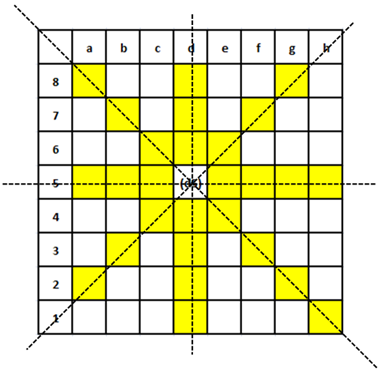### CAT 2017 Question Paper (Shift-2) - DILR Question 24

Instructions

In an 8 X 8 chess board a queen placed any where can attack another piece if the piece is present in the same row, or in the same column or in any diagonal position in any possible 4 directions, provided there is no other piece in between in the path from the queen to that piece.

The columns are labelled a to h (left to right) and the rows are numbered 1 to 8 (bottom to top). The position of a piece is given by the combination of column and row labels. For example, position c5 means that the piece is in $$c^{th}$$ column and $$5^{th}$$ row.

Question 24

# Suppose the queen is the only piece on the board and it is at position d5. In how many positions can another piece be placed on the board such that it is safe from attack from the queen?

SolutionFrom the diagram we can see that the number of positions those are safe from queen’s attack = 6 + 5 + 5 + 5 + 5 + 5 + 5 = 36. Therefore, option C is the correct answer.

OR